+

# Introduction to Parabolas

Author: Sophia Tutorial
##### Description:

Determine the vertex and direction of opening of the parabola for a quadratic equation.

(more)

Sophia’s self-paced online courses are a great way to save time and money as you earn credits eligible for transfer to many different colleges and universities.*

No credit card required

37 Sophia partners guarantee credit transfer.

299 Institutions have accepted or given pre-approval for credit transfer.

* The American Council on Education's College Credit Recommendation Service (ACE Credit®) has evaluated and recommended college credit for 32 of Sophia’s online courses. Many different colleges and universities consider ACE CREDIT recommendations in determining the applicability to their course and degree programs.

Tutorial
what's covered
1. Upwards and Downwards Parabolas
2. The Vertex of a Parabola
3. Vertex Form of a Quadratic Equation

# 1. Upwards and Downwards Parabolas

When graphing quadratic relationships, we can describe the curve on the coordinate plane as a parabola. Parabolas open either upwards or downwards, creating a U-shape curve (in the case of an upward parabola) or an upside down U-shape curve (in the case of a downwards parabola).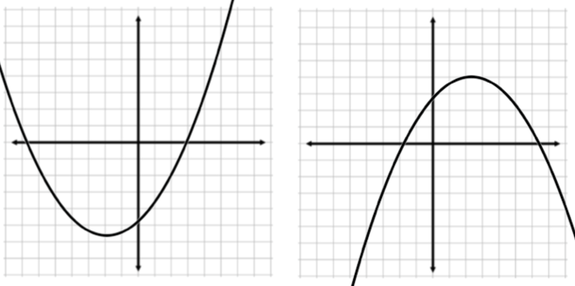One form of a quadratic equation is y = ax2 + bx + c (this is called standard form). The value of "a" dictates whether the parabola will open up or down. If a is a positive value, the parabola will open upwards. If a is a negative value, the parabola will open downwards.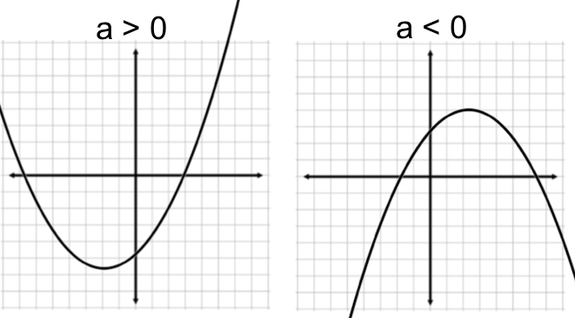# 2. The Vertex of a Parabola

Given the shape of a parabola, there will always be either a low point or a high point to the curve. This point is referred to as the parabola's vertex. An interesting characteristic of the vertex is that it lies on an invisible line of symmetry. This means that parabolas are symmetrical - we can imagine reflecting one side of the parabola about the line of reflection, and it will match up with points on the other side. This line of reflection is known as the axis of symmetry to the parabola.

terms to know
Vertex (of a parabola)
the minimum or maximum point of a parabola located on the axis of symmetry
Axis of Symmetry
a line of reflection passing through the vertex of a parabola; in up and down parabolas, it is a vertical line.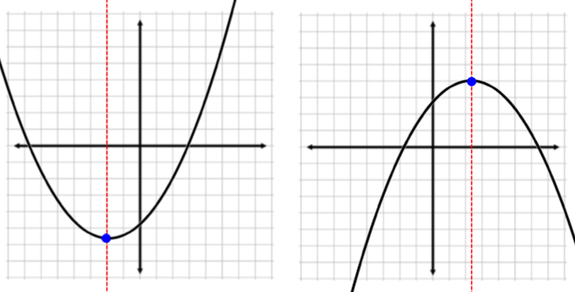There are a few ways to find the vertex of a parabola from its equation. When the equation of a parabola is given in standard form, we use the values a and b (the coefficients of the x-squared and x-terms) to calculate the x–coordinate of the vertex. This also describes the equation to the axis of symmetry. Here is the formula we use:

formula
x-coordinate of Vertex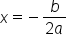Once we find the value for x, we plug that into the equation and find the associated y-value. This gives us the coordinates of the parabola's vertex.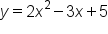Quadratic equation in standard form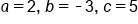Identify a, b, and c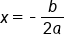Formula for the axis of symmetry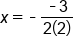Substitute for a and b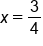x-coordinate of the vertex

Now we can use the value of 3/4, or 0.75, for x and solve for y to find the y–coordinate.Quadratic equation in standard form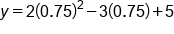x=0.75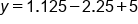Evaluate each term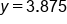y-coordinate of the vertex

The vertex tois located at the point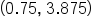# 3. Vertex Form of a Quadratic Equation

Finding the coordinates to a parabola's vertex is much easier if the equation in given in a different format, specifically the vertex form.

formula
Vertex Form of a Quadratic Equation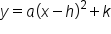We don't call it vertex form for nothing. The variables h and k represent the x– and y–coordinates of the vertex. So there is virtually no calculations needed in order to identify the vertex. The only tricky thing is to remember that in general, there is a minus sign between x and h, so we need to be mindful of the sign.

hint

It is easy to mistake the sign of h when finding the x–coordinate of the vertex. Because the general formula subtracts h from x, if we see a plus sign in the specific equation, our h value is actually negative.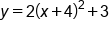Our equation in vertex form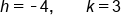Identify h and k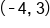Coordinates to the vertex

summary
A quadratic equation can either be an upwards or downwards parabola. If the x-squared coefficient in a quadratic equation is positive, the graph of the equation parabola will be pointing up. If the x-squared coefficient is negative, the parabola will be pointing down. The vertex of a parabola is either the low point or the high point to the curve. The axis of symmetry acts as a line of reflection, so all points on the left of the parabola can be reflected across the axis of symmetry to represent all points to the right and vice versa. With the vertex form of a quadratic equation, the variable h and k represent the x- and y-coordinates of the vertex.
Terms to Know
Axis of Symmetry

a line of reflection passing through the vertex of a parabola; in up and down parabolas, it is a vertical line.

Vertex (of a parabola)

the maximum or minimum point of a parabola located on the axis of symmetry

Formulas to Know
Vertex Form of a Quadratic EquationX-Coordinate of VertexRating